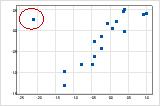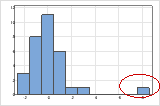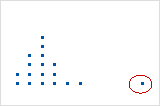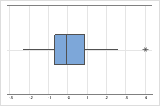# Interpret the key results for Marginal Plot

Complete the following steps to interpret a marginal plot.

## Step 1: Look for a model relationship and assess its strength

Determine which model relationship best fits your data and assess the strength of the relationship.

###### Tip

To better visualize how well a particular model fit suits your data, create a scatterplot with a fitted regression line.

### Type of relationship

Determine which model relationship, if any, best fits your data. The following are examples of the types of relationships.

If your data seem to fit a model, you can explore the relationship using a regression analysis.

### Strength of relationship

Assess how closely the data fit the model to estimate the strength of the relationship between X and Y. When the relationship is strong, the regression equation models the data accurately.

To quantify the strength of a linear (straight) relationship, use a correlation analysis.

## Step 2: Look for indicators of nonnormal or unusual data

Skewed data and multi-modal data indicate that data may be nonnormal. Outliers may indicate other conditions in your data.

### Skewed data

When data are skewed, the majority of the data are located on the high or low side of the graph. Skewness indicates that the data may not be normally distributed. In a marginal plot, look at the graphs in the margins for indicators of skewed data.

For example, the following graphs with right-skewed data show wait times. Most of the wait times are relatively short, and only a few wait times are long. The following graphs with left-skewed data show failure time data. A few items fail immediately, and many more items fail later.
Histograms
Dotplots
Boxplots

If you know that your data are not naturally skewed, investigate possible causes. If you want to analyze severely skewed data, read the data considerations topic for the analysis to make sure that you can use data that are not normal.

### Outliers

Outliers, which are data values that are far away from other data values, can strongly affect your results. In a marginal plot, look at the scatterplot and the graphs in the margins for outliers.

Scatterplot
On a scatterplot, isolated points identify outliers.Histogram
On a histogram, isolated bars at the ends identify outliers.Dotplot
On a dotplot, unusually low or high data values identify possible outliers.Boxplot
On a boxplot, outliers are identified by asterisks (*).Try to identify the cause of any outliers. Correct any data entry or measurement errors. Consider removing data values that are associated with abnormal, one-time events (special causes). Then, repeat the analysis.

### Multi-modal data

Multi-modal data have more than one peak. (A peak represents the mode of a set of data.) Multi-modal data usually occur when the data are collected from more than one process or condition, such as at more than one temperature.

In a marginal plot, look at the histograms or dotplots in the margins for indicators of multi-modal data. For example, these graphs have two peaks.

If you have additional information that allows you to classify the observations into groups, you can create a group variable with this information. Then, you can create a histogram or dotplot with groups to determine whether the group variable accounts for the peaks in the data.